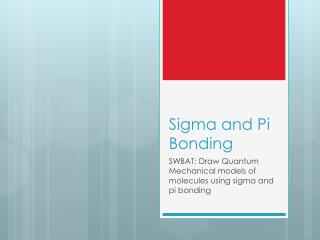# Sigma and Pi Bonding - PowerPoint PPT PresentationDownload PresentationSigma and Pi Bonding

Sigma and Pi BondingDownload Presentation## Sigma and Pi Bonding

- - - - - - - - - - - - - - - - - - - - - - - - - - - E N D - - - - - - - - - - - - - - - - - - - - - - - - - - -
##### Presentation Transcript

1. Sigma and Pi Bonding SWBAT: Draw Quantum Mechanical models of molecules using sigma and pi bonding

2. Carbon Hybridization

3. Carbon Hybridization

4. sp3 Hybridization

5. Carbon Hybridization

6. sp2 Hybridization • C2H4

7. Carbon Hybridization

8. sp Hybridization • C2H2

9. Covalent Bonds • The formation of a covalent bond involves the overlapping of half filled atomic orbitals • The two different categories of covalent bonds are Sigma and Pi covalent bonds

10. Sigma bonding • Bond is formed by the axial overlapping of half filled atomic orbitals • The electron cloud formed is cylindrically symmetrical about inter nuclear axis • The electrons constituting sigma bonds are called sigma electrons

11. 3 Types of Sigma Bonding • s-s overlap • s-p overlap • p-p overlap

12. sp-overlaps Example: CH4

13. Strength of Sigma Bonds • The strength of three types of sigma bonds varies as follows • p-p > p-s > s-s • A p-p overlap is greater than an s-s overlap allowing it to be stronger

14. Pi Bonding • This type of covalent bond is formed by the lateral or sidewise overlap of the atomic orbitals • The orbital overlap takes place in a way that their axes are parallel to each other but perpendicular to the internuclear axis • The pi bond consists of two charge clouds above and below the plane of the atoms involved in the bond formation • The electrons involved in the p bond formation are called pi electrons

15. Pi Bonding

16. Sigma and Pi Bonding • Sigma bonds are stronger than pi bonds (greater overlap between orbitals occurs) • Pi bonding between the two atoms is formed only in addition to a sigma bond (Sigma first then Pi) • Atoms rotate around sigma bonds but not pi bonds • The shape of the molecule is controlled by the orientations of sigma bonds around the central atom. Pi bonds are superimposed on sigma bonds.

17. Sigma and Pi bonding with Oxygen (O2) • 1s22s22p4 • s orbitals are filled and the 2px orbital is filled • The 2py and 2pz orbitals are partially filled • An O=O molecule has one sigma and one pi bond (connect both lobes of a p orbital for a pi bond…. Draw two lines for one pi bond)

18. Oxygen (O2) Nitrogen (N2)